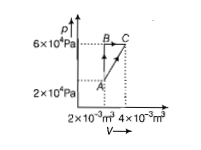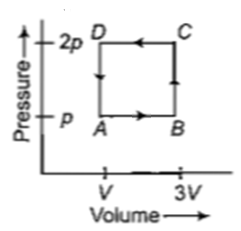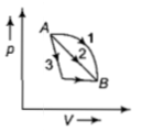You’ve reached the end of your free Videos limit.
#33 | Application of First Law to IsoThermal Process
(Physics) > Thermodynamics
Unable to watch the video, please try another server
Related Practice Questions :

A system is taken from state A to state B along two different paths 1 and 2. If the heat absorbed and work done by the system along these two paths are  respectively, then

(1) ${Q}_{1}={Q}_{2}$

(2) ${W}_{1}={W}_{2}$

(3) ${Q}_{1}-{W}_{1}={Q}_{2}-{W}_{2}$

(4) ${Q}_{1}+{W}_{1}={Q}_{2}+{W}_{2}$

High Yielding Test Series + Question Bank - NEET 2020

Difficulty Level:

Figure below shows two paths that may be taken by a gas to go from a state A to a state C. In process AB, 400 J of heat is added to the system and in process BC, 100 J of heat is added to the system. The heat absorbed by the system in the process AC will be-(a) 380 J
(b) 500 J
(c) 460 J
(d) 300 J

High Yielding Test Series + Question Bank - NEET 2020

Difficulty Level:

A thermodynamic system is taken through the cycle ABCD as shown in figure. Heat rejected by the gas during the cycle is(a)2 pV                                     (b)4 pV

(c)$\frac{1}{2}pV$                                    (d)pV

High Yielding Test Series + Question Bank - NEET 2020

Difficulty Level:

An ideal gas goes from state A to state B

via three different processes as indicated

in the p-V diagram

If  indicates the heat absorbed

by the gas along the three processes and

indicates the change in

internal energy along the three processes

respectively, then(a)

(b)

(c)

(d)

High Yielding Test Series + Question Bank - NEET 2020

Difficulty Level:

The internal energy change in a system that has absorbed 2 kcal of heat and done 500 J of work is

(a) 8900 J                                      (b) 6400 J

(c) 5400 J                                      (d) 7900 J